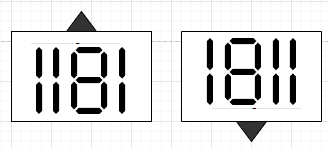# Check whether N is a Dihedral Prime Number or not in Python

Suppose we have a number n. We have to check whether n is dihedral prime or not. A number is said to be dihedral prime when that number is itself prime and also shown same number or any other prime number using 7-segment display regardless the orientation of the display (normal or up-side down).

So, if the input is like n = 1181, then the output will be Truesecond one is up-side down format of the first one and both are prime.

To solve this, we will follow these steps −

• Define a function up_side_down() . This will take n
• temp := n, total := 0
• while temp > 0, do
• d := temp mod 10
• if d is same as 2, then d := 5
• otherwise when d is same as 5, then d := 2
• total := total * 10 + d
• temp := quotient of (temp / 10)
• From the main method do the following:
• if n is not prime or up_side_down(n) is not prime or reverse of n is not prime or reverse of up_side_down(n) is not prime, then
• return False
• temp := n
• while temp > 0, do
• rem := temp mod 10
• if rem is any of these [3, 4, 6, 7, 9], then
• return False
• temp := quotient of (temp / 10)
• return True

Let us see the following implementation to get better understanding −

## Example Code

Live Demo

prime = (int(1e5)+5)*[True]
def reverse(n):
return int(str(n)[::-1])

def up_side_down(n):
temp = n
total = 0
while temp>0:
d = temp % 10
if d == 2:
d = 5
elif d == 5:
d = 2
total = total * 10 + d
temp//= 10

def get_all_prime():
prime = prime = False

for i in range(2, int(1e5)+1):
j = 2
while i * j<= int(1e5):
prime[i * j] = False
j+= 1

def solve(n):
get_all_prime()
if not prime[n] or not prime[up_side_down(n)] or not prime[reverse(n)] or not prime[reverse(up_side_down(n))]:
return False

temp = n

while temp>0:
rem = temp % 10;
if rem in [3, 4, 6, 7, 9]:
return False
temp //= 10

return True

n = 1181
print(solve(n))



## Input

23, 3

## Output

True